• 这是款使用CSS3 animation动画和png图片制作的css3线条波浪动画效果。该特效中，使用了3张波浪线条的png图片，以及少量的CSS代码，制作出逼真的线条波浪动画效果。CSS3库
• title author date CreateTime ... WPF 如何给定两个点画出一条波浪线 lindexi 2019-6-27 10:17:6 +0800 2019-6-26 14:36:5 +0800 WPF 在知道两个点可以连一条线段，那么将线段修改...

titleauthordateCreateTimecategories

WPF 如何给定两个点画出一条波浪线

lindexi

2019-6-27 10:17:6 +0800

2019-6-26 14:36:5 +0800

WPF

在知道两个点可以连一条线段，那么将线段修改为波浪线可以如何做？
在知道两个点 p1 和 p2 的时候，按照这两个点画出一条波浪线要求波浪线的中间就在两个点连接的线段
我做了一个程序用于显示效果，这个程序的界面用到很好用的 HandyControl 库可以尝试设置一些参数画出好看的线，这个程序全部开源，可以在github下载全部源代码，核心源代码将会放在本文后面
下面告诉大家如何在知道两个点的时候画出一条波浪线
在开始之前需要小伙伴熟悉贝塞尔的概念如果使用控制点画出贝塞尔，使用本文的方法画出的波浪线会比直接连出线段的投影长度短一点，但是看起来几乎相同
贝塞尔曲线可以通过两个点和一个锚点控制一段曲线，我将两个点连接一段线段在这段线段上均匀放置一些锚点，在线段的上下方分别放控制点，通过一个锚点和两个控制点可以绘制出一段贝塞尔线。请看图绿色的点是控制点，黑色的点是锚点刚好所有的锚点都在一条线段上，通过锚点和控制点就可以画出曲线将控制点和锚点链接作为贝塞尔的效果如下图这里用到了两个控制点和一个锚点画贝塞尔，这里需要用到 Path 绘制的特性。在 Path 绘制的时候将会不断记住上一个点的值，也就是我输入的点其实会影响到上一条绘制命令的点如在我已经知道了两个控制点和一个锚点组成的数组。我每次调用 streamGeometryContext.BezierTo 的时候都需要传入三个点，第一个点控制点用来控制前一个命令的锚点而第二个点也是控制点用于控制本次调用方法里面的锚点也就是第三个点从上面可以知道绘制曲线会受到很多常量的影响，这些常量建议作为用户定义。为了让大家能和代码关联起来，先定义一些变量，如增幅和频率等，可以下载我的代码运行一下，自己修改这些值知道了曲线是如何画的，现在的问题就是如何求出这两个控制点和一个锚点的值
本文将会用到 bcp 算法，在说到用两个点绘制波浪线的时候，小伙伴最先想到的是使用水平线，但实际上任意两个点很少是水平的，也就是需要将波浪线绘制在一个协办上，于是如下图定义了摆角和斜角这里的 bcpInclination 是摆角表示波形的高度，这个值配合频率可以决定波形的高度，反过来知道频率和高度就可以计算出这个值
另外还可以了斜角 wiggle angle 表示给出的两点连出的线段与水平的夹角
现在尝试计算一下摆角的值，在计算摆角的值之前需要先拿到频率和高度从图片可以看到三角形 abc 的两个值，如 a 的值就是四分之一的频率，三角形的 b 的值就是波形的高度的一半

a = wave length / 4
b = wave height / 2

这里的频率其实和波形长度是相同的，计算方法是在输入两个点的时候，计算出线段的长度

var distance = (p1 - p2).Length;

通过波形的长度计算出这段长度有多少个波形

var waveLength = WaveLength;
var howManyWaves = distance / waveLength;

于是反过来计算波形的频率

var waveInterval = distance / howManyWaves;

此时简化数学计算，可以知道 waveInterval 就是波形的长度
于是按照向量的计算方法可以知道摆角的值可以使用下面代码计算

var bcpInclination = CalculateAngle(new Point(0, 0), new Point(a, b));

上图的三角形的 c 边就是定义的 max bcp length 的值，表示在当波形是三角形时的长度用三角形的计算方法，在知道 a 和 b 的时候求 c 边的长度可以使用下面方法

var maxBcpLength =
Math.Sqrt(waveInterval / 4.0 * (waveInterval / 4.0) + waveHeight / 2.0 * (waveHeight / 2.0));

其实上面的代码只是模拟计算而已，代码可以继续优化
在知道了 maxBcpLength 可以通过一个常量知道控制点距离锚点的距离如上图可以知道 bcpLength 的值就是控制点距离锚点的距离
刚好 bcpLength 就是最大长度的某个比例，可以通过下面代码计算

var bcpLength = maxBcpLength * curveSquaring;

上面代码的 curveSquaring 是一个常量可以给用户定义，用于控制波形实际高度与给定高度的关系
在知道了控制点距离锚点的长度，需要求当前控制点的坐标需要再知道两个值，一个是某个端点的坐标另一个是线段的切斜角度
刚好控制点的一个端点就是锚点，也就是链接锚点和控制点成为的线段刚好就是控制点距离锚点的长度，那么先求锚点对应的值
求锚点的值可以转换为求某线段上，均匀分多个点，求这些点的坐标的计算方法
如果这条线段是水平线段，那么很好求，于是先假设线段是水平的求出均匀的点，通过知道三角形的两个角度和一条边可以计算出另一条边的长度，请看图和代码这部分比较简单var flexPt = new Point(prevFlexPt.X + Math.Cos(angle) * waveInterval / 2.0,
prevFlexPt.Y + Math.Sin(angle) * waveInterval / 2.0);

上面代码的 prevFlexPt 就是上一个锚点的坐标，而 flexPt 就是当前锚点的坐标，第一个锚点就是线段的一个端点
现在第二个计算是倾斜角，请看下图可以知道控制点的倾斜角可以知道倾斜角和摆角相关，在知道锚点的坐标和距离和倾斜角就可以计算出控制点的坐标，在知道控制点和锚点就可以画出曲线
这是核心代码

var p1 = startPoint;
var p2 = endPoint;

var distance = (p1 - p2).Length;

var angle = CalculateAngle(p1, p2);
var waveLength = WaveLength;
var waveHeight = WaveHeight;
var howManyWaves = distance / waveLength;
var waveInterval = distance / howManyWaves;
var maxBcpLength =
Math.Sqrt(waveInterval / 4.0 * (waveInterval / 4.0) + waveHeight / 2.0 * (waveHeight / 2.0));

var curveSquaring = CurveSquaring;
var bcpLength = maxBcpLength * curveSquaring;
var bcpInclination = CalculateAngle(new Point(0, 0), new Point(waveInterval / 4.0, waveHeight / 2.0));

var wigglePoints = new List<(Point bcpOut, Point bcpIn, Point anchor)>();
var prevFlexPt = p1;
var polarity = 1;

for (var waveIndex = 0; waveIndex < howManyWaves * 2; waveIndex++)
{
var bcpOutAngle = angle + bcpInclination * polarity;
var bcpOut = new Point(prevFlexPt.X + Math.Cos(bcpOutAngle) * bcpLength,
prevFlexPt.Y + Math.Sin(bcpOutAngle) * bcpLength);
var flexPt = new Point(prevFlexPt.X + Math.Cos(angle) * waveInterval / 2.0,
prevFlexPt.Y + Math.Sin(angle) * waveInterval / 2.0);
var bcpInAngle = angle + (Math.PI - bcpInclination) * polarity;
var bcpIn = new Point(flexPt.X + Math.Cos(bcpInAngle) * bcpLength,
flexPt.Y + Math.Sin(bcpInAngle) * bcpLength);

polarity *= -1;
prevFlexPt = flexPt;
}

var streamGeometry = new StreamGeometry();
using (var streamGeometryContext = streamGeometry.Open())
{
streamGeometryContext.BeginFigure(wigglePoints.anchor, true, false);

for (var i = 1; i < wigglePoints.Count; i += 1)
{
var (bcpOut, bcpIn, anchor) = wigglePoints[i];

streamGeometryContext.BezierTo(bcpOut, bcpIn, anchor, true, false);
}
}

private static double CalculateAngle(Point p1, Point p2)
{
return Math.Atan2(p2.Y - p1.Y, p2.X - p1.X);
}

上面代码用到一些属性

public double WaveLength { get; set; } = 4;
public double WaveHeight { get; set; } = 5;

public double CurveSquaring { get; set; } = 0.57;

这个类的代码在 WaveLine.cs
本文参考了 How to draw a wiggle between two points with Python and Drawbot - Medium

展开全文• Java波浪文字制作方法及源代码 1个目标文件 摘要:Java源码,初学实例,波浪文字 Java波浪文字，个利用Java处理字符的实例，可以设置运动方向参数，显示文本的字符数组，高速文本颜色，显示字体的 FontMetrics对象，...java
• 很简单，先找到设置，然后搜索 python.linting 然后选择下面的那个内容，取消勾选就行了 对，另外推荐vs code中python的编辑的个插件，名字叫 kite，下面是下载网站
很简单，先找到设置，然后搜索 python.linting 然后选择下面的那个内容，取消勾选就行了对，另外推荐vs code中python的编辑的一个插件，名字叫 kite，下面是下载网站https://kite.com/
展开全文• 搜了圈,基本都是建议使用drawArc方法画圆弧拼接波浪线,但是及其麻烦,效果如下,并不理想 在群里问了下大佬的建议,改变思路 那么接下来,开始研究下怎么画 1.已知条件,是飞机的两个点 先画出直线看看 ...
 需求是根据飞机的线路情况,需要画直线,双直线,波浪线,虚线,连连接航路
其他都好说,这波浪线尤其不好画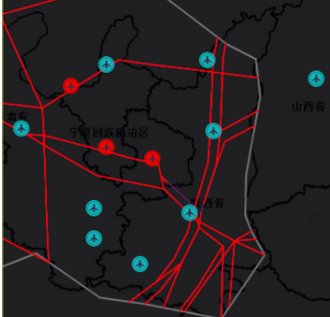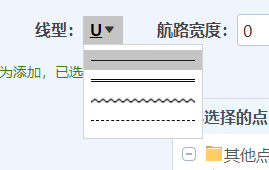搜了一圈,基本都是建议使用drawArc方法画圆弧拼接波浪线,但是及其麻烦,效果如下,并不理想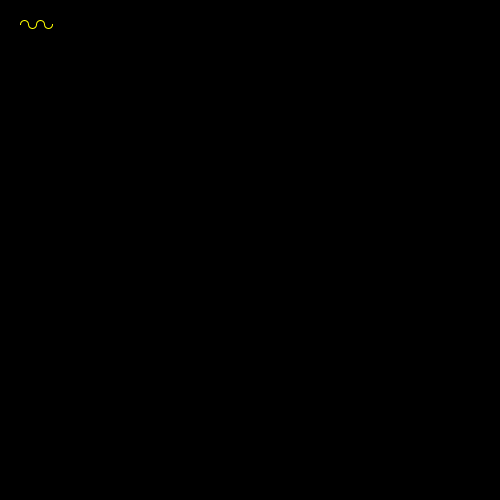在群里问了下大佬的建议,改变思路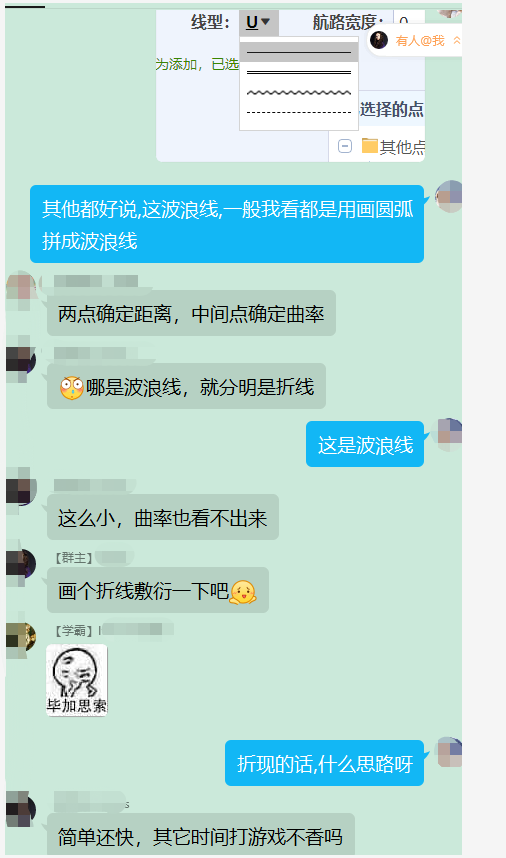那么接下来,开始研究下怎么画
1.已知条件,是飞机的两个点
先画出直线看看
代码:
 public static void main(String[] args) throws IOException {
BufferedImage res = null;
res = new BufferedImage(500, 500,
BufferedImage.TYPE_INT_RGB);
Graphics2D g = res.createGraphics();
g.setRenderingHint(RenderingHints.KEY_ANTIALIASING, RenderingHints.VALUE_ANTIALIAS_ON);
g.setBackground(Color.black);
g.clearRect(0, 0, 500, 500);

int x1=200,y1=100;
int x2=400,y2=400;
g.drawLine(x1,y1,x2,y2);

String imageDir = "D:\\出图测试\\波浪";

ImageInfoUtil.write(res, imageDir, "test.png");

}
public static void write(BufferedImage subimage, String centerImageDir, String fileName) throws IOException {
FileOutputStream pointOut = null;
try {
File f = new File(centerImageDir);
if(!f.isDirectory()){
f.mkdirs();
}
pointOut = new FileOutputStream(centerImageDir+File.separator+fileName);//输出图片的地址
ImageIO.write(subimage, "png", pointOut);
}finally {
if(pointOut!=null){
pointOut.close();
}
}
}
效果: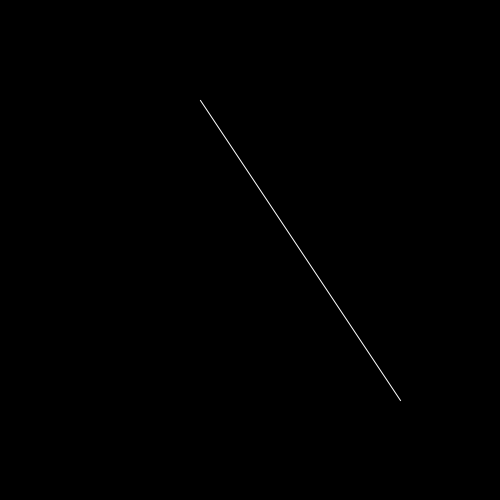2.根据直线求平行线
草稿: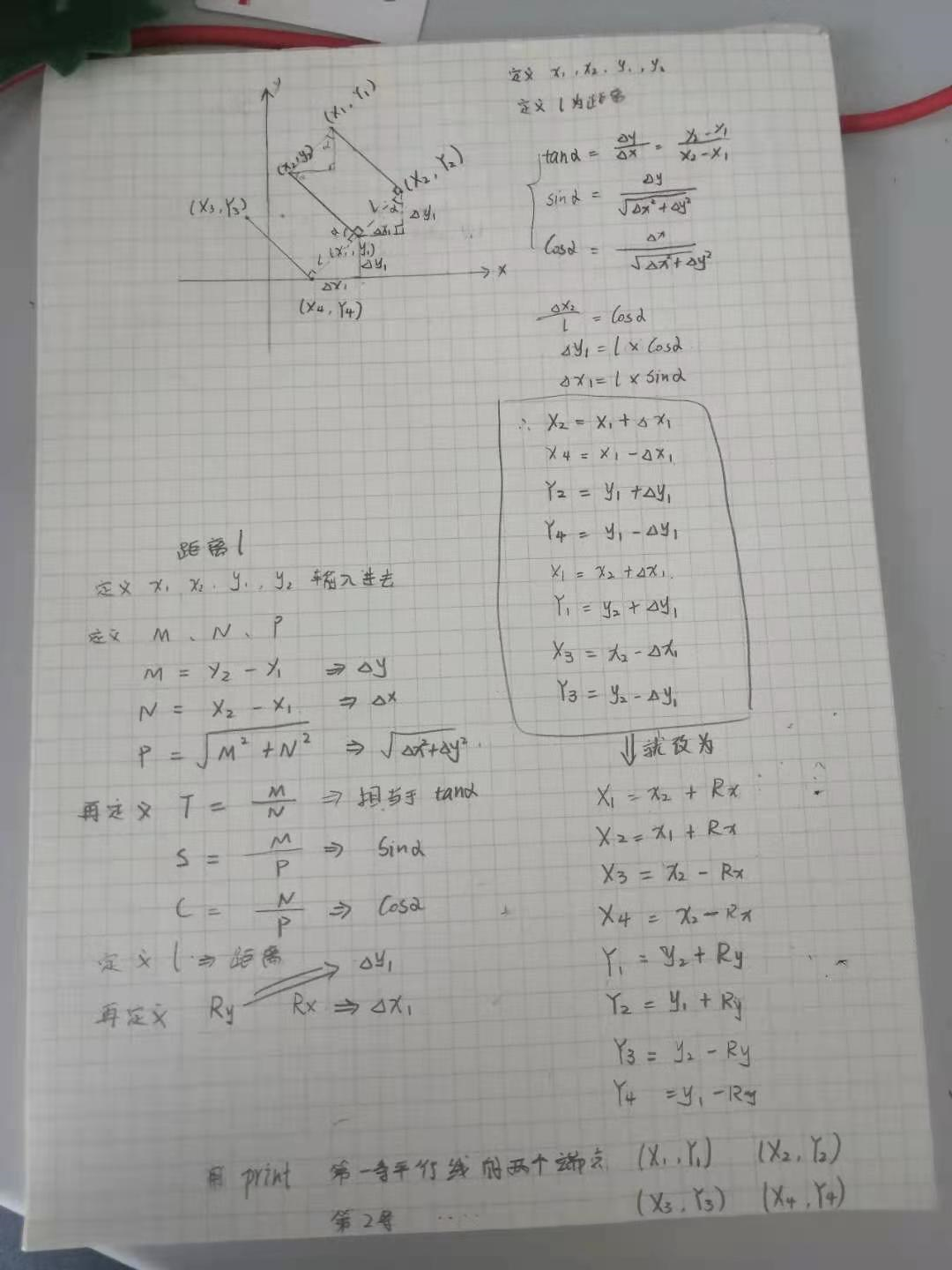代码:
public static void main(String[] args) throws IOException {
BufferedImage res = null;
res = new BufferedImage(500, 500,
BufferedImage.TYPE_INT_RGB);
Graphics2D g = res.createGraphics();
g.setRenderingHint(RenderingHints.KEY_ANTIALIASING, RenderingHints.VALUE_ANTIALIAS_ON);
g.setBackground(Color.black);
g.clearRect(0, 0, 500, 500);

int x1=200,y1=100;
int x2=400,y2=400;
g.drawLine(x1,y1,x2,y2);

int[] ps= parallelLines(x1,y1,x2,y2,3);
g.setColor(Color.green);
g.drawLine(ps,ps,ps,ps);
g.drawLine(ps,ps,ps,ps);

String imageDir = "D:\\出图测试\\波浪";

ImageInfoUtil.write(res, imageDir, "test.png");

}
public static void write(BufferedImage subimage, String centerImageDir, String fileName) throws IOException {
FileOutputStream pointOut = null;
try {
File f = new File(centerImageDir);
if(!f.isDirectory()){
f.mkdirs();
}
pointOut = new FileOutputStream(centerImageDir+File.separator+fileName);//输出图片的地址
ImageIO.write(subimage, "png", pointOut);
}finally {
if(pointOut!=null){
pointOut.close();
}
}
}

/**
* 根据两点一直线和距离,算出两天平行线
* @param x1
* @param y1
* @param x2
* @param y2
* @param l
* @return
*/
public static int[] parallelLines(int x1, int y1, int x2, int y2,int l) {
int m = y2-y1;
int n = x2-x1;
double p = Math.sqrt(Math.pow(m, 2) + Math.pow(n, 2));
double t = p/n;
double s=m/p;
double c = n/p;
double ry=l*c;
double rx=l*s;

int x3 = (int) (x2-rx);
int x4=(int)(x1-rx);

int y3 = (int) (y2+ry);
int y4=(int)(y1+ry);

int x5 = (int) (x2+rx);
int x6=(int)(x1+rx);

int y5 = (int) (y2-ry);
int y6=(int)(y1-ry);

return new int[]{x3,y3,x4,y4,x5,y5,x6,y6};
}
效果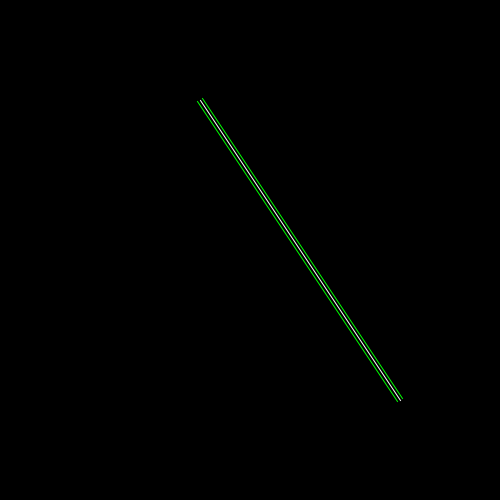3.以已知两点为首尾,两平行线上取点,进行连接为折线(需要用到直线公式计算斜率这不贴草稿了)
代码:稍微封装了一下

public class DTest {
public static void main(String[] args) throws IOException {
BufferedImage res = null;
res = new BufferedImage(500, 500,
BufferedImage.TYPE_INT_RGB);
Graphics2D g = res.createGraphics();
g.setRenderingHint(RenderingHints.KEY_ANTIALIASING, RenderingHints.VALUE_ANTIALIAS_ON);
g.setBackground(Color.black);
g.clearRect(0, 0, 500, 500);

int x1=200,y1=100;
int x2=400,y2=400;
g.drawLine(x1,y1,x2,y2);

int[] ps= CoordinateUtil.parallelLines(x1,y1,x2,y2,3);
g.setColor(Color.green);
g.drawLine(ps,ps,ps,ps);
g.drawLine(ps,ps,ps,ps);

g.setColor(Color.red);

int x3 = ps;
int y3 = ps;
int x4 = ps;
int y4 = ps;
int[] y34=null;
int[] x34=null;

int x5 = ps;
int y5=  ps;
int x6 = ps;
int y6 = ps;
int[] y56=null;
int[] x56=null;

if(x1==x2){
y34 = CoordinateUtil.numbers(y3,y4);
x34 = new int[y34.length];
for (int i = 0; i < x34.length; i++) {
x34[i]=x3;

}
y56 = CoordinateUtil.numbers(y5,y6);
x56 = new int[y56.length];
for (int i = 0; i < x56.length; i++) {
x56[i]=x5;
}
}else{

x34 = CoordinateUtil.numbers(x3,x4);
double k34=CoordinateUtil.K(y3,y4,x3,x4);
double b34=CoordinateUtil.B(y3,x3,k34);
y34 = CoordinateUtil.pointY(x34,k34,b34);

x56 = CoordinateUtil.numbers(x5,x6);
double k56=CoordinateUtil.K(y5,y6,x5,x6);
double b56=CoordinateUtil.B(y5,x5,k56);
y56 = CoordinateUtil.pointY(x56,k56,b56);
}

CoordinateUtil.XY[] xy34=new  CoordinateUtil.XY[y34.length];
CoordinateUtil.XY[] xy56=new  CoordinateUtil.XY[y56.length];
for (int i = 0; i < y34.length; i++) {
xy34[i] = new CoordinateUtil.XY(x34[i],y34[i]);
}

for (int i = 0; i < y56.length; i++) {
xy56[i]=new CoordinateUtil.XY(x56[i],y56[i]);
}

CoordinateUtil.XY[] polys = CoordinateUtil.polyXYs(new CoordinateUtil.XY(x1,y1),new CoordinateUtil.XY(x2,y2),xy34,xy56,3);

int[]  polyX = new int[polys.length];
int[]  polyY = new int[polys.length];

for (int i = 0; i < polys.length; i++) {
polyX[i]=polys[i].x;
polyY[i]=polys[i].y;
}

g.drawPolyline(polyX, polyY, polyX.length);

String imageDir = "D:\\出图测试\\波浪";

ImageInfoUtil.write(res, imageDir, "test.png");

}
public static void write(BufferedImage subimage, String centerImageDir, String fileName) throws IOException {
FileOutputStream pointOut = null;
try {
File f = new File(centerImageDir);
if(!f.isDirectory()){
f.mkdirs();
}
pointOut = new FileOutputStream(centerImageDir+File.separator+fileName);//输出图片的地址
ImageIO.write(subimage, "png", pointOut);
}finally {
if(pointOut!=null){
pointOut.close();
}
}
}

}

public class CoordinateUtil {

/**
* 根据两个值,获取中间所有数
* 包含首尾
* @param x1
* @param x2
* @return
*/
public static int[] numbers(int x1, int x2) {
int max = Math.max(x1, x2);
int min = Math.min(x1, x2);
int index=0;
int [] px = new int[max-min+1];
for (int i = min; i <= max; i++) {
px[index]=i;
index++;
}
return px;
}

/**
* 求常量B
* @param y
* @param x
* @param k
* @return
*/
public static double B(int y, int x, double k) {
double b = new BigDecimal(String.valueOf(y)).subtract(new BigDecimal(new BigDecimal(String.valueOf(k)).multiply(new BigDecimal(String.valueOf(x))).doubleValue())).doubleValue();
return b;
}

/**
* 求斜率k
* @param y1
* @param y2
* @param x1
* @param x2
* @return
*/
public static double K(int y1, int y2, int x1, int x2) {
if(x2 - x1==0){
throw new RuntimeException("x2与x1相等,没有斜率");
}
double k = new BigDecimal(String.valueOf(y2 - y1)).divide(new BigDecimal(String.valueOf(x2 - x1)),5, BigDecimal.ROUND_HALF_UP).doubleValue();
return k;
}

/**
* 根据两点一直线和距离,算出两天平行线
* @param x1
* @param y1
* @param x2
* @param y2
* @param l
* @return
*/
public static int[] parallelLines(int x1, int y1, int x2, int y2,int l) {
int m = y2-y1;
int n = x2-x1;
double p = Math.sqrt(Math.pow(m, 2) + Math.pow(n, 2));
double t = p/n;
double s=m/p;
double c = n/p;
double ry=l*c;
double rx=l*s;

int x3 = (int) (x2-rx);
int x4=(int)(x1-rx);

int y3 = (int) (y2+ry);
int y4=(int)(y1+ry);

int x5 = (int) (x2+rx);
int x6=(int)(x1+rx);

int y5 = (int) (y2-ry);
int y6=(int)(y1-ry);

return new int[]{x3,y3,x4,y4,x5,y5,x6,y6};
}

/**
* 根据中间两点取首位点
* @param xy1 首
* @param xy2 尾
* @param xy34 平行线1
* @param xy56 平行线2
* @param interval 波浪间隔
* @return
*/
public static XY[] polyXYs(XY xy1, XY xy2, XY[] xy34, XY[] xy56,int interval) {
if(xy34.length!= xy56.length){
throw  new RuntimeException("两条平行线长度应相同");
}
List<XY> xyList = new ArrayList<>();
int lg=1;
for (int i = interval; i < xy34.length; i+=interval) {
if(i>xy34.length){
break;
}
if(lg%2==1){
}else{
}
lg++;
}
return xyList.toArray(new XY[xyList.size()]);
}

public static class XY{
public int x;
public int y;

public XY(int x, int y) {
this.x = x;
this.y = y;
}
}

/**
* y=kx+b
* @param px
* @param k
* @param b
* @return
*/
public static int[] pointY(int[] px, double k, double b) {
int[] py = new int[px.length];
for (int i = 0; i < px.length; i++) {
}
return py;
}
}
效果: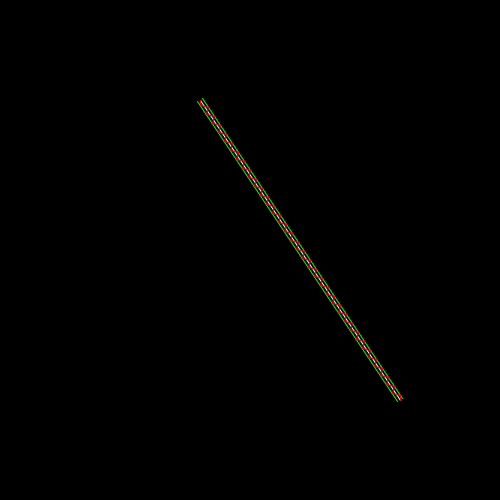去掉辅助的平行线和直线看看,对比一下效果还不错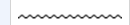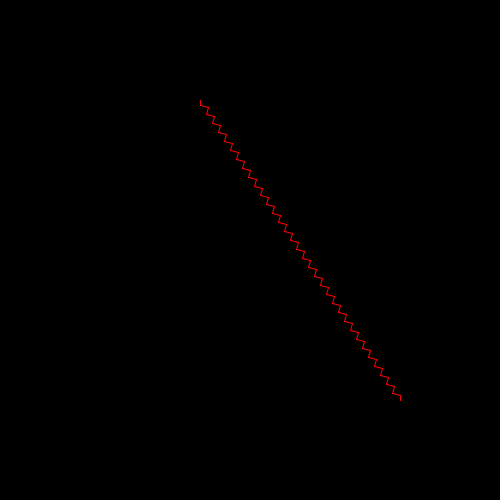代码未整理封装,有空再说


展开全文java Java画图
• xml bitmap是个用xml定义的文件放在资源目录，定义的对象是图片，为bitmap定义别名，这个文件可以给bitmap定义一些额外的属性。例如：抖动。1、文件存放位置res/drawable/filename.xml2、语法?例子:?效果图：默认...

我们要实现的效果如下：在这之前先带大家了解一下xml bitmap，何为xml bitmap？
xml bitmap是一个用xml定义的文件放在资源目录，定义的对象是图片，为bitmap定义别名，这个文件可以给bitmap定义一些额外的属性。例如：抖动。
1、文件存放位置
res/drawable/filename.xml
2、语法
?
例子:
?
效果图：
默认(@drawable/btn_default_pressed_holo_light)：引用(\@drawable/bm)：从截图上就可以看出来差别。
titilemode="repeat":之后找了一些关于实现波浪线的方法，总感觉不大满意，常见的方法分享给大家：
1、直接搞一个这样的波浪线的切图
这种方式最简单，但是劣势也非常明显，如果view的宽度过大，则会出现图片变形，如下图所示：如果过小则如下：要求不高的话，这样勉强可以蒙混过关，但是追求完美的话，这样的效果显然很不给力
2、自定义控件绘制
这个方法的确可以达到不错的效果，但是实现比较麻烦。我也看到有朋友搞过，
3、用xml的bitmap标签实现波浪线效果
首页要搞一个周期的波浪线，即包括波峰和波谷，如下图所示：
wave_item:然后水平方向上平铺，从而实现波浪线效果,代码如下：
wave.xml：
?
activity_main.xml
?
注意：这个imageview通过background显示图片，src是显示不了这样的效果的。
希望本文所述对大家学习android软件编程有所帮助。

展开全文• // 从最左侧开始, 画到最右侧, 每20px画一条线, // 这里注意是 (), 改成 for (int x = 0; x (); x += 20) { // float y = A sin (ωx + φ) + k; float y1 = A * (float) Math.sin(ω * x + φ) + A; float y2...
• 不知道各位发现没有，将其他地方的文字段落复制到Word2013中时，会有很多蓝色红色的波浪线。这是语法错误引起的，具体是什么语法在此就不做讨论，下面，主要是来介绍怎么快速去除这些波浪线。 ①启动Word2013，将...Word
• 如果你需要绘制波浪线，只要连续输入三个或者三个以上的“～” ，然后回车，波浪线就自动生成了；如果你需要绘制虚线，只要连续输入三个或者三个以上的“*” ，然后回车，虚线就自动生成了；如果你需
• 本次的分享是个基于canvas的更新图片特效实现。其中主要涉及canvas中getImageData()、putImageData()、toDataURL()方法的使用。效果请看下面。 欢迎关注我的博客，不定期更新中—— PS:请在本地服务器中打开页面，...javascript 前端 canvas
• ## 消除PyCharm中满屏的波浪线

万次阅读 多人点赞 2016-08-24 14:55:13
PyCharm使用了较为严格的PEP8的检查规则，如果代码命名不规范，甚至多出的空格都会被波浪线标识出来，导致整个编辑器里铺满了波浪线，右边的滚动也全是黄色或灰色的标记线，很是影响编辑，这里给大家分享一下代码...PyCharm Python
• 我们在读纸质书的时候，经常用笔划波浪线来突出重点内容，以强化视觉感受。 而在Web页面上，没有CSS3之前，我们只能用背景图片来实现类似功能，这当然不是个高效的方式，且难于维护和调整。 比如你只是想调整下颜色...CSS3 线性渐变 下划线
• * 进度条终点图片 * */ private Drawable progressDrawable; /** * 振幅 */ private float amplitude = 1 ; /** * 音量 */ private float volume = 10 ; private int fineness = ...android 动画
• 去掉讨厌的小波浪线 1.设置背景图片 想像我一样写代码的时候有美女相伴？像这样 最爱的紫霞仙子！！！ Pycharm里就可以设置：File --> Settings --> Appearance&Behavior --> Appearance 再按红色框...
• 写这篇文章的初衷是因为早些时候看到款morning routine上的窗帘皱褶效果，自己也想去实现它，网上也有一些案例但是效果不太好而且没有任何的注释改动难度比较，因此想通过自己的想法去实现这个效果，让后面的人少...扭曲
• ## JAVA上百实例源码以及开源项目

千次下载 热门讨论 2016-01-03 17:37:40
Java波浪文字，个利用Java处理字符的实例，可以设置运动方向参数，显示文本的字符数组，高速文本颜色，显示字体的 FontMetrics对象，得到Graphics实例，得到Image实例，填充颜色数组数据，初始化颜色数组。...
• 　PowerPoint2013并没有提供波浪线的形状，如果我们要使用PPT提供的线条去绘制的话会比较麻烦，没有基础的人...　1、启动PPT，在幻灯片插入个文本框，我们利用微软拼音输入法打出波浪线(微软拼音输入法做的比国内好
• 由于公司改变了SDK的样式要求，波浪线的绘制就到这里，不过今后随着我学习深入会补充随高度变化的水波纹效果以及更绚丽的效果。 public class View1 extends View { Context context ; Paint linePaint1; Paint ...android
• 段时间，web端的同事想实现个海浪进度条，但是他不知道怎么去实现，我这个人比较热心，就...原理其实很简单了，不过就是画贝塞尔曲线，然后不停的来回移动画好的图片，造成波浪滚动的假象，再调调高度，波浪...Flutter 进度条 Bar
• 我：波浪效果是吧，小意思，个月的奶茶就够了，或者扫码提需求~ ????美术小姐姐：皮？???????????? ????我：卒~ 俗话说：遇事不决，量子力学写虽得儿。 根据我多年喝奶茶的经验，像这种效果用 Shader 做就Shader 游戏开发
• 首先分析一下我的做法，我是将波浪的部分和头像分开考虑，根据波浪的移动高度将头像画出 、定义属性 其实我在做的时候我是直接开始画，画完了才去优化自定义属性，然而现在这些过程已经不重要了，我就先android
• 之前3.5版本 没有问题，更新到4.1后出现红波浪，但不影响运行。并且文件不爆红，看不见问题所在。哪位大神，解答一下，谢谢 <p style="text-align:center"><img alt="" height="35" src=...
• 大家好，我是深红骑士，爱开玩笑，技术渣渣，热爱钻研，这篇文章是今年的最后篇了，首先祝大家在新的一年里心想事成，诸事顺利。今天来学习贝塞尔曲线，之前一直想学，可惜没时间。什么是贝塞尔曲线呢？开始我...
• 波浪线 粗细线 多头线 图形 游戏流程图 入职流程图 日记流程图 矩形和线条，可以展现一切 箭头试试：如何用矩形和线条表达这句话？ 武松是潘金莲的搭档1.没有箭头graph LR A[武松]---|搭档|B[潘金莲]这种没有箭头...有道云笔记
• 这是一张由正弦曲线组成的波浪曲面，在U和V两个方向上，每一条相邻的正弦曲线都是由一条正弦曲线平移得到，懂了吧。 画正弦曲线，只需要找到正弦曲线上的几个关键点，然后将关键点连接即可。 ......